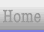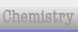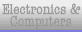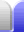### Lowest Common Denominator

To be able to compare two fractions (or add them together, or subtract one from the other) they need to have the same denominator.

We know that multiplying by 1 won't change the value of a number, so if we multiply a fraction by a fraction equal to 1 it won't change in value. There are lots to chose from, and we can pick any we like.

 1 = 1⁄1 = 2⁄2 = 3⁄3 = ... = 6,782 = ...6,782

Let's look at an example. Assuming you're hungry, would you rather have 29 of an ice cream cake or 524 of it? To be sure of which is bigger we'll have to convert them so they have the same denominator. The easiest way to choose one is to use the denominator of the other fraction.

29 x 2424 = 48216

524 x 99 = 45216

So 29 > 524 by 3216

But 216 is divisible by 3 (because it's 63 and 6 is divisible by 3, and because 2+1+6 is a multiple of 3) which means there were common factors in the denominators. In this case, both 9 and 24 can be divided by 3. Finding the lowest common multiple of the original two denominators will give us the lowest common denominator, which will give us the simplest fraction at the end of it.

29 x 88 = 1672

524 x 33 = 1572

So 29 > 524 by 172

172 of an ice cream cake isn't much, but it's still worth having.

"Lowest common denominator" is also used figuratively to describe how television sometimes creates their programmes to appeal to the lowest ideals of their viewing audience, for example by using the simplest, coarsest humour, instead of anything that might require careful consideration.

To be able to add two fractions together they need the same denominator. If the denominators are not the same we need to make them the same by the method mentioned above. Let's look at an example.

13 + 14 = ( 13 x 44 ) + ( 14 x 33 ) = 412 + 312

Once the denominators are the same the numerators can simply be added together while the denominator stays the same.

412 + 312 = 712

As I mentioned earlier, sometimes the denominators will have common factors. For example:

12 + 14 = ( 12 x 44 ) + ( 14 x 22 ) = 48 + 28 = 68

Then simplify 68 = 34

Or we could use a better multiplying fraction to start with, so we can use the lowest common denominator.

12 + 14 = ( 12 x 22 ) + 14 = 24 + 14 = 34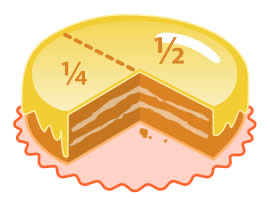The ice cream cake example above was actually an example where we subtracted one fraction from another.

29 - 524 = 1672 - 1572 = 172Home Astronomy Chemistry Electronics & Computers Mathematics Physics Field Trips Turn on javascript for email link